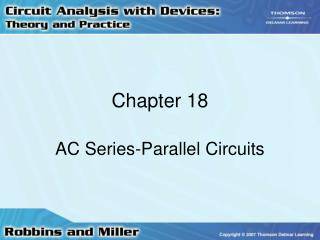DownloadDownload PresentationChapter 18

# Chapter 18

Download Presentation## Chapter 18

- - - - - - - - - - - - - - - - - - - - - - - - - - - E N D - - - - - - - - - - - - - - - - - - - - - - - - - - -
##### Presentation Transcript

1. Chapter 18 AC Series-Parallel Circuits

2. AC Circuits • Rules and laws developed for dc circuits apply equally well for ac circuits • Analysis of ac circuits requires vector algebra and use of complex numbers • Voltages and currents in phasor form • Expressed as RMS (or effective) values

3. Ohm’s Law • Voltage and current of a resistor will be in phase • Impedance of a resistor is: ZR = R0°

4. Ohm’s Law • Voltage across an inductor leads the current by 90°(ELI the ICE man)

5. Ohm’s Law • Current through a capacitor leads the voltage by 90° (ELI the ICE man)

6. AC Series Circuits • Current everywhere in a series circuit is the same • Impedance used to collectively determine how resistance, capacitance, and inductance impede current in a circuit

7. AC Series Circuits • Total impedance in a circuit is found by adding all individual impedances vectorially

8. AC Series Circuits • Impedance vectors will appear in either the first or the fourth quadrants because the resistance vector is always positive • When impedance vector appears in first quadrant, the circuit is inductive

9. AC Series Circuits • If impedance vector appears in fourth quadrant • Circuit is capacitive

10. Voltage Divider Rule • Voltage divider rule works the same as with dc circuits • From Ohm’s law:

11. Kirchhoff’s Voltage Law • KVL is same as in dc circuits • Phasor sum of voltage drops and rises around a closed loop is equal to zero

12. Kirchhoff’s Voltage Law • Voltages • May be added in phasor form or in rectangular form • If using rectangular form • Add real parts together • Then add imaginary parts together

13. AC Parallel Circuits • Conductance, G • Reciprocal of the resistance • Susceptance, B • Reciprocal of the reactance

14. AC Parallel Circuits • Admittance, Y • Reciprocal of the impedance • Units for all of these are siemens (S)

15. AC Parallel Circuits • Impedances in parallel add together like resistors in parallel • These impedances must be added vectorially

16. AC Parallel Circuits • Whenever a capacitor and an inductor having equal reactances are placed in parallel • Equivalent circuit of the two components is an open circuit

17. Kirchhoff’s Current Law • KCL is same as in dc circuits • Summation of current phasors entering and leaving a node • Equal to zero

18. Kirchhoff’s Current Law • Currents must be added vectorially • Currents entering are positive • Currents leaving are negative

19. Current Divider Rule • In a parallel circuit • Voltages across all branches are equal

20. Series-Parallel Circuits • Label all impedances with magnitude and the associated angle • Analysis is simplified by starting with easily recognized combinations

21. Series-Parallel Circuits • Redraw circuit if necessary for further simplification • Fundamental rules and laws of circuit analysis must apply in all cases

22. Frequency Effects of RC Circuits • Impedance of a capacitor decreases as the frequency increases • For dc (f = 0 Hz) • Impedance of the capacitor is infinite

23. Frequency Effects of RC Circuits • For a series RC circuit • Total impedance approaches R as the frequency increases • For a parallel RC circuit • As frequency increases, impedance goes from R to a smaller value

24. Frequency Effects of RL Circuits • Impedance of an inductor increases as frequency increases • At dc (f = 0 Hz) • Inductor looks like a short • At high frequencies, it looks like an open

25. Frequency Effects of RL Circuits • In a series RL circuit • Impedance increases from R to a larger value • In a parallel RL circuit • Impedance increases from a small value to R

26. Corner Frequency • Corner frequency is a break point on the frequency response graph • For a capacitive circuit • C = 1/RC = 1/ • For an inductive circuit • C = R/L = 1/

27. RLC Circuits • In a circuit with R, L, and C components combined in series-parallel combinations • Impedance may rise or fall across a range of frequencies • In a series branch • Impedance of inductor may equal the capacitor

28. RLC Circuits • Impedances would cancel • Leaving impedance of resistor as the only impedance • Condition is referred to as resonance

29. Applications • AC circuits may be simplified as a series circuit having resistance and a reactance • AC circuit • May be represented as an equivalent parallel circuit with a single resistor and a single reactance

30. Applications • Any equivalent circuit will be valid only at the given frequency of operation